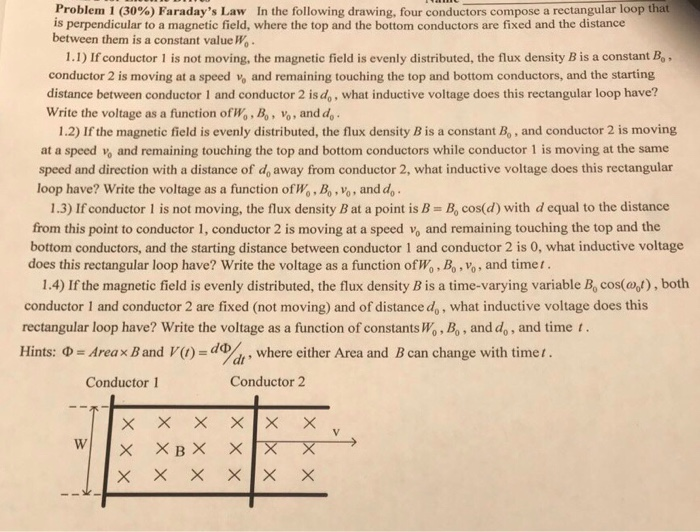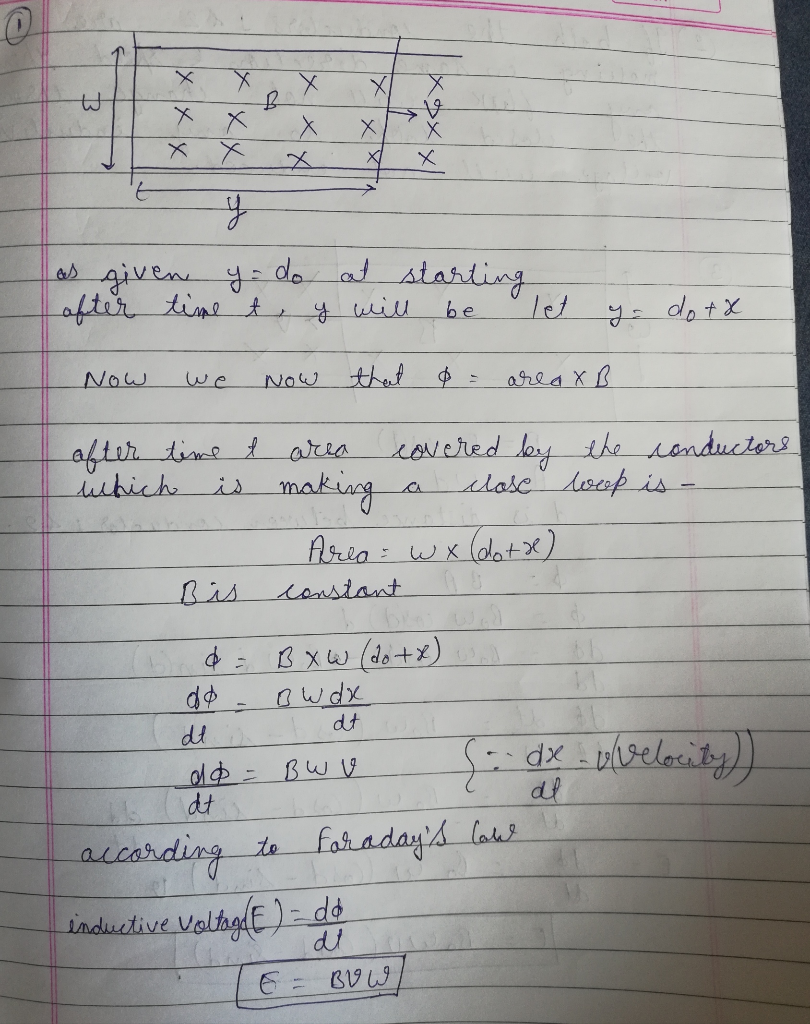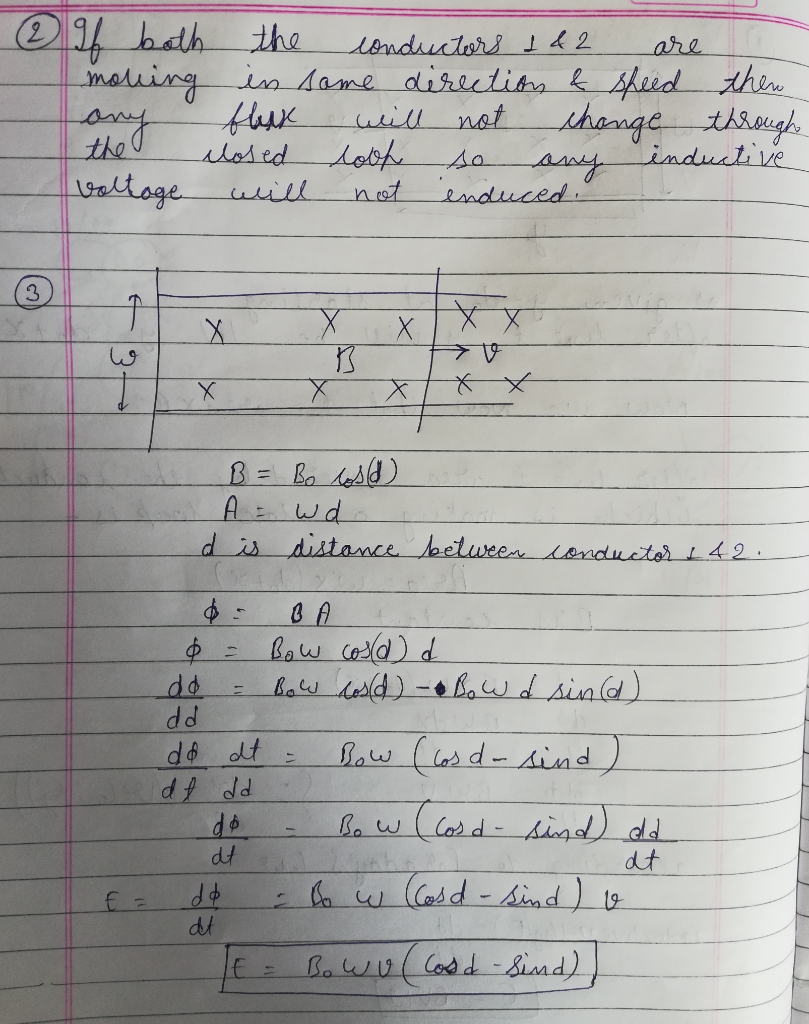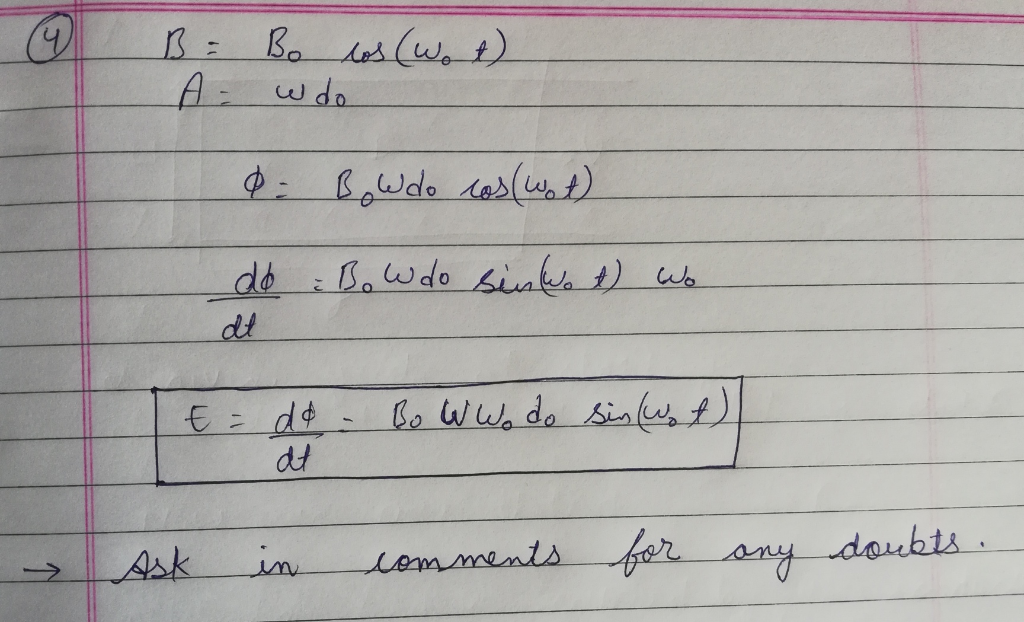# Please answer everything with steps. Thank you Problem 1(30%)Faraday's Law In the following drawing, four conductors compose a rectangular loop that is perpendicular to a magnetic field, wh...Problem 1(30%)Faraday's Law In the following drawing, four conductors compose a rectangular loop that is perpendicular to a magnetic field, where the top and the bottom conductors are fixed and the distance between them is a constant valueWo 1.1) If conductor 1 is not moving, the magnetic field is evenly distributed, the flux density B is a constant Bo conductor 2 is moving at a speed vo and remaining touching the top and bottom conductors, and the starting distance between conductor 1 and conductor 2 is do, what inductive voltage does this rectangular loop have? Write the voltage as a function ofWo, Bo, vo and do 1.2) If the magnetic field is evenly distributed, the flux density B is a constant Bo, and conductor 2 is moving at a speed v% and remaining touching the top and bottom conductors while conductor 1 is moving at the same speed and direction with a distance of d, away from conductor 2, what inductive voltage does this rectangular 1.3) If conductor 1 is not moving, the flux density B at a point is B- B, cos(d) with d equal to the distance bottom conductors, and the starting distance between conductor 1 and conductor 2 is 0, what inductive voltage loop have? Write the voltage as a function of Wo, Bo, vo, and d from this point to conductor 1, conductor 2 is moving at a speed vo and remaining touching the top and the does this rectangular loop have? Write the voltage as a function ofWo, Bo, vo, and time t 1.4) If the magnetic field is evenly distributed, the flux density B is a time-varying variable B, cos(oy), both conductor 1 and conductor 2 are fixed (not moving) and of distance do, what inductive voltage does this rectangular loop have? Write the voltage as a function of constants Wo, B,, and do, and time t. Hints: Φ = Areax8 and V() = da , where either Area and B can change with time 1 . Conductor 1 Conductor 2##### Add Answer of: Please answer everything with steps. Thank you Problem 1(30%)Faraday's Law In the following drawing, four conductors compose a rectangular loop that is perpendicular to a magnetic field, wh...
More Homework Help Questions Additional questions in this topic.